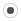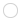## How to create sparse diagonal matrix efficientlymaolijian
Registered MemberPosts
2
Karma
0

### How to create sparse diagonal matrix efficiently

Wed Feb 27, 2019 3:20 am
Hello,
I have a large vector D ,when I want to use
Code: Select all
`D.asDiagonal();`
, it occured memory error.
So I change a new way to create sparse diagonal matrix
Code: Select all
`1: SparseMatrix<double> mat(rows,cols);         // default is column major2: mat.reserve(VectorXi::Constant(cols,6));3: for each i,j such that v_ij != 04:   mat.insert(i,j) = v_ij;                    // alternative: mat.coeffRef(i,j) += v_ij;5: mat.makeCompressed();                        // optional`

But I found it was too slow.
I want to know how to imrove it ?
Thank you!annayu
Registered MemberPosts
1
Karma
0

### Re: How to create sparse diagonal matrix efficiently

Sat Mar 09, 2019 7:03 am
Use scipy.sparse.spdiags (which does a lot, and so may be confusing, at first), scipy.sparse.dia_matrix and/or scipy.sparse.lil_diags. (depending on the format ttrockstars login you want the sparse matrix in...)

E.g. using spdiags:

Code: Select all
`import numpy as npimport scipy as spimport scipy.sparsex = np.arange(10)# "0" here indicates the main diagonal...# "y" will be a dia_matrix type of sparse array, by default y = sp.sparse.spdiags(x, 0, x.size, x.size)`ballen
Registered MemberPosts
3
Karma
0

### Re: How to create sparse diagonal matrix efficiently

Wed Mar 13, 2019 11:22 amdarrellj
Registered MemberPosts
1
Karma
0

### Re: How to create sparse diagonal matrix efficiently

Wed Apr 03, 2019 6:52 am
I would like to solve a PDE using an implicit finite volume discretization. Since the problem is 3D, the matrix should be septadiagonal. I have computed the 7 diagonals (for top, bottom, east, west, north south and central point) in 7 different arrays. dqfanfeedback

## Who is online

Registered users: alainroger, Arkengheist, Baidu [Spider], berndmj, Bing [Bot], claydoh, gi1242, Google [Bot], jmacleod, kde-cgvirus, rigobot, thetimbrick, wolfn, Yahoo [Bot]